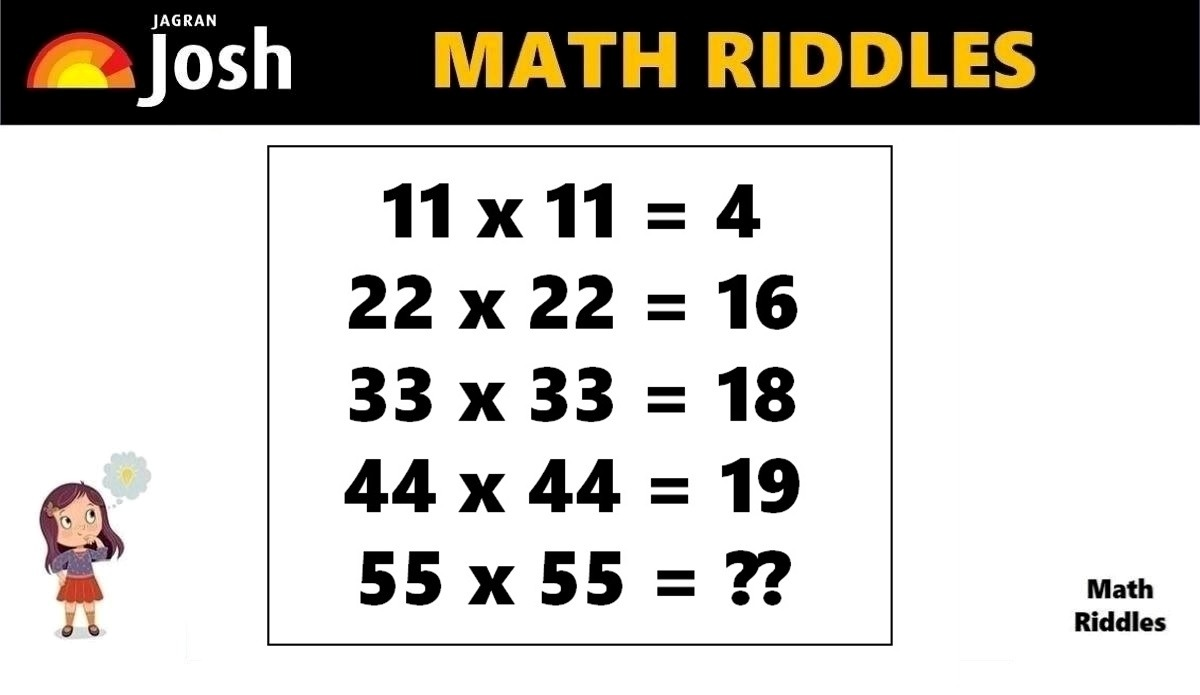# Math Riddles With Answers: Only Mathematics Genius Can Solve This Puzzle in 20 Seconds

In this Math Puzzle, we need to find the logic in each equation to find the solution. Only mathematics experts can solve this logical mathematics problem within 20 seconds.Math Riddles With Answers: Only Mathematics Genius Can Solve This Puzzle in 20 Seconds

In this Math Puzzle, we need to find the logic in each equation to find the solution. There are 5 logic-based maths equations in this math riddle. All math enthusiasts, teachers, students, and learners of any age group can try to solve this puzzle. As we know Mathematics is a tough subject so let us make Maths fun and interactive.

Are you a math genius? Only 1% with a high IQ can solve this tricky math puzzle in 20 seconds.

Share this math puzzle with your friends, kids, students, colleagues, and family. Time to test your mathematical knowledge and IQ level with this math puzzle. Let us solve this math picture puzzle within 20 seconds.

If you love solving cryptic math puzzles, give this math puzzle a try! You have 20 seconds to find the correct answer.

## Math Riddles with Answers: Solution

Explanation:

Let us summarise all the equations below and give them reference point as below:

11 x 11 = 4 …………… Equation 1

22 x 22 = 16 ………….. Equation 2

33 x 33 = 18 ………….. Equation 3

44 x 44 = 19 ………….. Equation 4

55 x 55 = ?? ………………. Equation 5

Only 1% Genius Can Find The Hidden Words In This Living Room Within 11 Seconds

Let us start solving the equations. We shall perform the operation Multiplication as given to see the actual products of each equation.

=> 11 x 11 = 121

=> 22 x 22 = 484

=> 33 x 33 = 1089

=> 44 x 44 = 1936

Only 1% Visually Intelligent Can Spot The Toilet Paper Roll Within 11 Seconds

Now, we shall add up each digit of all the products in each equation. Let us see if get the desired results. See below,

=> 11 x 11 = 121 = 1 + 2 + 1 = 4

=> 22 x 22 = 484 = 4 + 8 + 4 = 16

=> 33 x 33 = 1089 = 1 + 0 + 8 + 9 = 18

=> 44 x 44 = 1936 = 1 + 9 + 3 + 6 = 19

Math Riddles With Answers: Solve This Donald Duck, Jerry, Mickey Mouse Math Puzzle in 20 Seconds

Hence, we figured out the logic. We shall apply the same logic to find the solution for Equation 5.

=> 55 x 55 = 3025

=> 55 x 55 = 3025 = 3 + 0 + 2 + 5 = 10

Great Work!

## Tell us in comments: Did you solve this math puzzle correctly in 20 seconds?

Check out more amazing tricky math puzzles!

Math Riddles With Answers: Can You Find The Value of Red Fish and Blue Fish in 20 Seconds?

Get the latest General Knowledge and Current Affairs from all over India and world for all competitive exams.
खेलें हर किस्म के रोमांच से भरपूर गेम्स सिर्फ़ जागरण प्ले पर